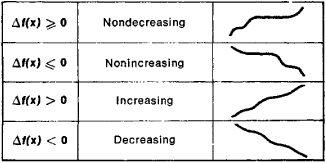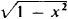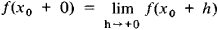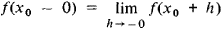Monotonic Function

(redirected from Strictly increasing)

monotonic function

[¦män·ə¦tän·ik ′fəŋk·shən]
(mathematics)

Monotonic Function

(or monotone function), a function whose increments Δf(x) = f(x′) − f(x) do not change sign when Δx = x′ − x > 0; that is, the increments are either always nonnegative or always nonpositive. Somewhat inaccurately, a monotonic function can be defined as a function that always varies in the same direction. Different types of monotonic functions are represented in Figure 1. For example, the function y = x3 is an increasing function. If a function f(x) has a derivative f′(x) that is nonnegative at every point and that vanishes only at a finite number of individual points, then f(x) is an increasing function. Similarly, if f′(x) ≤ 0 and vanishes only at a finite number of points, then f(x) is a decreasing function.Figure 1

A monotonicity condition can hold either for all x or for x on a given interval. In the latter case, the function is said to be monotonic on this interval. For example, the function y =increases on the interval [−1,0] and decreases on the interval [0, +1]. A monotonic function is one of the simplest classes of functions and is continually encountered in mathematical analysis and the theory of functions. If f(x) is a monotonic function, then the following limits exist for any X0:andReferences in periodicals archive ?
rho] < [kappa]] is strictly increasing and cofinal in C.
is strictly increasing with h and g is increasing it follows that
Stokey, Lucas, and Prescott, 1989), our assumptions on u ensure that the autarky value function [OMEGA](a) is continuously differentiable, strictly increasing, and strictly concave for all a [member of] A.
Gregoriades examines the equivalence classes under effective Borel isomorphism, between complete separable metric spaces that admit a recursive presentation, and show the existence of strictly increasing and strictly decreasing sequences as well as of infinite anti-chains under the natural notion of effective isomorphism reduction, as opposed to the non-effective case, where only two such classes exist, the one of the Baire space and the one of the naturals.
p](a, b) are continuous and strictly increasing with respect to p [member of] R for fixed a,b > 0 with a [not equal to] b.
Note that for v [less than or equal to] 30, u(v) given by (3) can be viewed as a strictly increasing function of v, we therefore have
n,k]([lambda], c) (for a fixed value of k and n > 0) is a strictly increasing function of [lambda].
Assumption 2 The belief function f(t) is strictly increasing.
1], is given by arranging the entries from each column of a hook composition tableau U so that the unprimed entries are strictly increasing from bottom to top, and above them the primed entries are weakly increasing from bottom to top.
It is assumed that the DM's value function is pseudo concave, and is strictly increasing in input with a maximal value at MPS.
A mapping T is called strongly [phi]-pseudocontractive if for all x, y [member of] C, there exist j(x--y) [member of] J(x - y) and a strictly increasing function [phi] : [0, [infinity]) [right arrow] [0, [infinity]) with [PHI](0) = 0 such that

Site: Follow: Share:
Open / Close